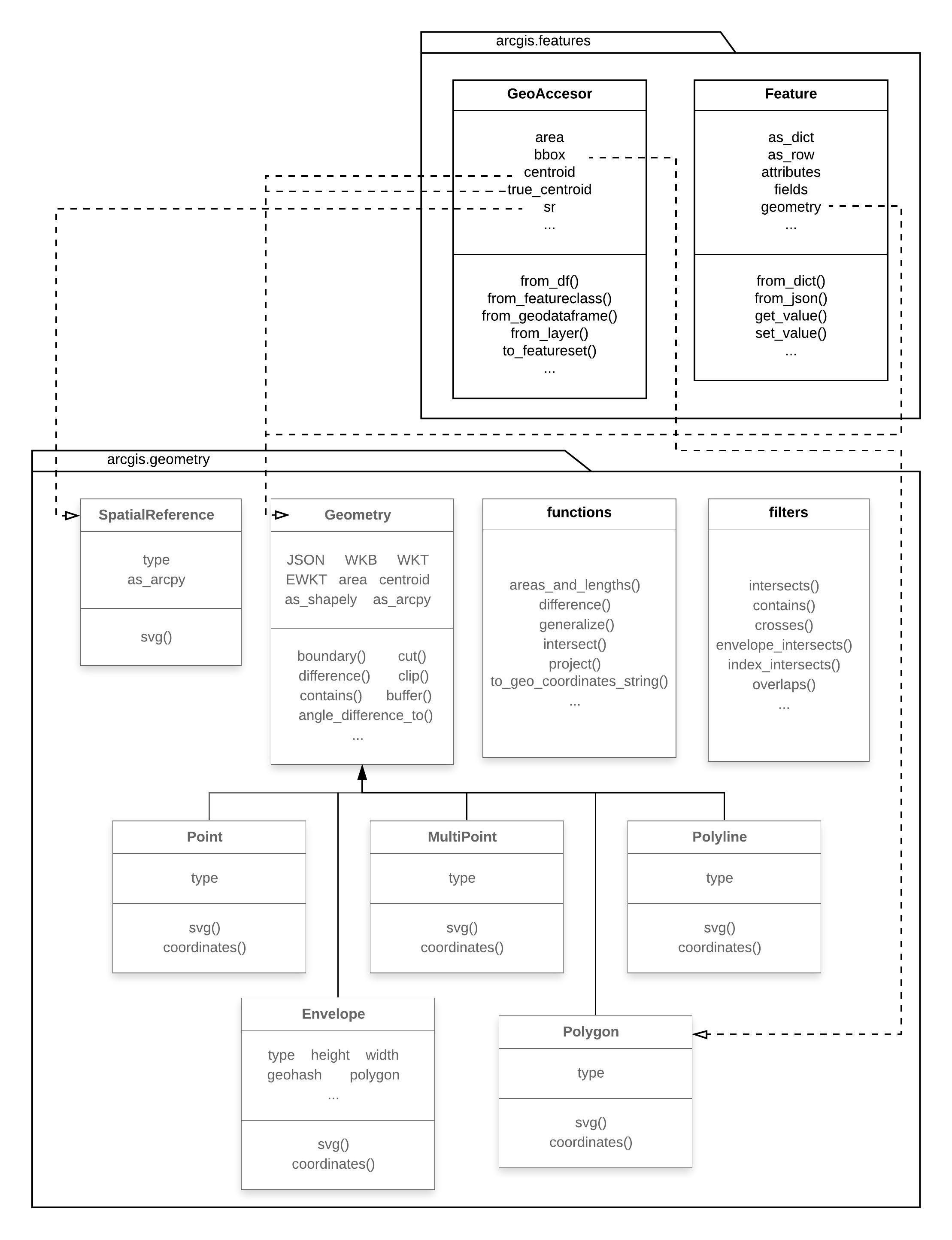ArcGIS Developers
Dashboard

# Part 2 - Working with Geometries¶

## Creating geometries¶

The `arcgis.geometry` module defines geometry types for working with geographic features in a GIS. It provides functions which use geometric types as input and output as well as functions for easily converting geometries between different representations.

Several functions in this module accept geometries represented as Python dictionary objects. To get started, we import the following classes and functions from the `geometry` module.

In :
```from arcgis.gis import GIS
from arcgis.geocoding import geocode
from arcgis.geometry import lengths, areas_and_lengths, project
from arcgis.geometry import Point, Polyline, Polygon, Geometry
import pandas as pd
```
In :
```gis = GIS('home')
```

### Object Model Diagram (OMD) of the geometry module¶

The picture below illustrates how the geometry module is organized (which showcase only the important objects, sub-modules, and properties via the OMD):The `arcgis.geometry` module contains classes and utility functions to construct geometry objects such as `Point`s, `Polyline`s, `Polygon`s and perform spatial operations on them. The `arcgis.geometry.Geometry` class is a base class from which specific geometry classes inherit. Users generally do not instantiate the base class, but work directly with one or more child classes such as `Point`, `Polyline`, `Polygon` etc. The base class provides geometry operations such as `clip()`, `difference()`, `buffer()`... which the child classes inherit. The `arcgis.geometry.functions` sub-module contains an alternate set of spatial operations. There difference between these two sets of operations is explained in the section 'Two patterns of applying spatial operations'.

The `SpatialReference` class is used to represent `spatial reference` and `coordinate system information`. The `arcgis.geometry.filters` module provides functions that can be used to define spatial relationships to be used in queries against feature layers and imagery layers.

The layout diagram further shows how `Feature` and `spatially enabled DataFrame` objects from `arcgis.features` module return components of the geometry module.

### Creating `Point` objects¶

A point contains x and y fields along with a `spatialReference` field. A point can also contain m and z fields as well, representing the vertical and linear referencing system coordinates. A point is empty when its x field is present and has the value null or the string `NaN`. An empty point has no location in space.

In :
```pt = Point({"x" : -118.15, "y" : 33.80,
"spatialReference" : {"wkid" : 4326}})
pt
```
Out:

As shown above, you can create a `Point` geometry using a dictionary. The `x` and `y` key value pairs in this example contain longitude and latitude respectively. The `spatialReference` dictionary with the `wkid` kvp specifies the coordinate system in which `x` and `y` are in. Thus, you could have passed it X and Y values from a projected coordinate system and constructed the same point by specifying the appropriate `wkid`.

When using the Jupyter Notebook (or ArcGIS Notebook) interface, you can query a geometry and get a visual representation as shown in the cell earlier. Alternately you can check the validity of a geometry by querying the `is_valid()` method.

In :
```pt.is_valid()
```
Out:
`True`
In :
```print(pt.is_empty)
```
```False
```

To get the geometry type, use the `type` property:

In :
```print(pt.type)
```
```Point
```

To get the object type in Python, use the built-in `type` function:

In :
```type(pt)
```
Out:
`arcgis.geometry._types.Point`
In :
```map0 = gis.map("Port of Long Beach")
map0.basemap = "satellite"
map0.zoom = 6
map0
```